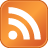## SUPPORT THE SITE WITH A CLICK

Subscribe Rss:# Ruby

A dynamic, open source programming language with a focus on simplicity and productivity. It has an elegant syntax that is natural to read and easy to write.

Some of the samples which in found in net for converting a Array to Hash

## Aray to Hash

`require "enumerator"class Array  def to_h    Hash[*enum_with_index.to_a.flatten]  endend%w{a b c d}.to_h  # =>  {"a"=>0, "b"=>1, "c"=>2, "d"=>3}`

`class Array  def to_h(keys)    Hash[*keys.zip(self).flatten]  endenda=['1','2','3']b=['4','5','6']a.to_h(b)=>{"6"=>"3", "4"=>"1", "5"=>"2"}`

`a = [1, 2, 3]Hash[*a.collect { |v| [v, v*2]}.flatten]=> {1=>2, 2=>4, 3=>6}`

`class << Hash  def create(keys, values)    self[*keys.zip(values).flatten]  endend>> Hash.create(['a', 'b', 'c'], [1, 2, 3])=> {"a"=>1, "b"=>2, "c"=>3}`

#### 2 comments :

1.a = [1,2,3]
( 0...a.size ).to_a.zip( a ).inject({}) { |h,x| h[x]=x; h }

2.thanks for ur reply